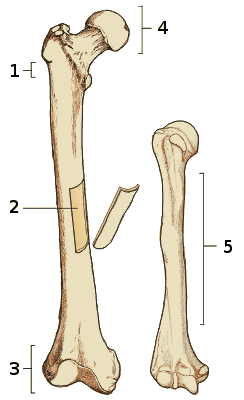15 questions on the anatomy of bones :This-quiz-uses-javascript!

The quiz above includes the following questions :

• Label number 1 in the diagram indicates which part of the bone?
• The bone on the left in the image is the :
• Label number 5 in the diagram indicates the :
• What do we mean by an 'articulation'?
• A 'crest' on a bone is :
• What is label number 4 pointing to in the diagram?
• What is a 'condyle'?
• A 'canal' is :
• Label number 3 in the diagram is pointing to :
• The bone on the right in the image is the :
• An 'articular process' is :
• In the diagram, what is label number 2 pointing to?
• A small projection or bump is known as an :
• What is the name for a projection near to a condyle?
• A small, flattened articular surface is called a :